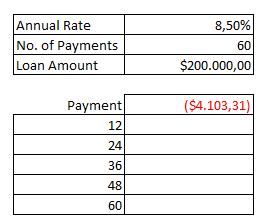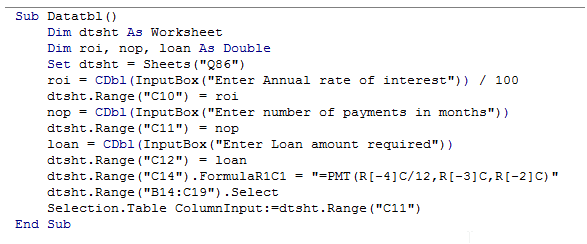## Vba data table in vba in Excel

The Data table is similar to the Scenario Manager and Goal Seek and does the same prediction function.

A data table is a range of cells in which you can change values in some of the cells and come up with different answers to a problem

There are two types of Data tables; One Variable data table and Two variable data table.

For illustration purpose, we will consider the One-variable data table.

One-variable data table is used while analyzing how different values of one variable in one or more formulas will change the results of those formulas.

The following example illustrates the use of Data table.

Let us build a data table that shows the monthly payments for loan terms ranging from 1 to 6 years.The Cells C10 to C12 contains the Loan details.

The following code shows how to create a datatable in the range B14:C19

1. Sub Datatbl()
2. Dim dtsht As Worksheet
3. Dim roi, nop, loan As Double
4. Set dtsht = Sheets("Q86")
5. roi = CDbl(InputBox("Enter Annual rate of interest")) / 100
6. dtsht.Range("C10") = roi
7. nop = CDbl(InputBox("Enter number of payments in months"))
8. dtsht.Range("C11") = nop
9. loan = CDbl(InputBox("Enter Loan amount required"))
10. dtsht.Range("C12") = loan
11. dtsht.Range("C14").FormulaR1C1 = "=PMT(R[-4]C/12,R[-3]C,R[-2]C)"
12. dtsht.Range("B14:C19").Select
13. Selection.Table ColumnInput:=dtsht.Range("C11")
14. End Sub

When executed, the program will demand the user to input Rate of Interest, Payment period and Loan amount.

Once entered, the program will create a datatable in the range B14:C19, corresponding to the values in B14:B19.

The screenshot of the editor is as shown below.